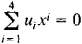# Projective Space

Also found in: Wikipedia.

## projective space

[prə′jek·tiv ′spās]
(mathematics)
The topological space obtained from the n-dimensional sphere under identification of antipodal points.

## Projective Space

in its original meaning, Euclidean space with the addition of the plane at infinity. Points at infinity, lines at infinity, and the plane at infinity are called ideal elements. In projective space, one ideal point is added to each line, one ideal line is added to each plane, and one ideal plane is added to the entire space. Parallel lines are supplemented by a common ideal point, and nonparallel lines by distinct ideal points. Parallel planes are supplemented by a common ideal line, and nonparallel planes by distinct ideal lines. The ideal points added to all the lines of a given plane belong to the ideal line added to this plane. All the ideal points and lines belong to the ideal plane.

Projective space can be analytically defined as the set of classes of proportional quadruples of real numbers that do not simultaneously equal zero. The classes can be interpreted as the points of projective space, in which case the numbers in the quadruples are called the homogeneous coordinates of the points. The classes can also be viewed as the planes of the projective space, in which case the numbers are called the homogeneous coordinates of the planes. The relation of incidence for a point (x1: x2: x3: x4) and a plane (u1: u2: u3: u4) is expressed by the equationThe concept of an n-dimensional projective space, which plays an important role in algebraic geometry, can be introduced in an analogous fashion. The coordinates of such a space are the elements of some skew field k. In the most general sense, a projective space is a collection of three sets of elements, called points, lines, and planes, for which the relations of membership and order are defined in such a way that the axioms of projective geometry are satisfied. A. N. Kolmogorov and L. S. Pontriagin have proved that if a projective space over a skew field k is a connected compact topological space, in which a line depends continuously on two of its points, and if the incidence axioms are satisfied, then k is the field of real numbers, the field of complex numbers, or the skew field of quaternions.

References in periodicals archive ?
A projective variety is a complex variety embedded in a projective space. By an endomorphism f: X [right arrow] X, we mean a morphism from a projective variety X to itself.
First of all, we take X to be the quaternionic projective space H[P.sub.n] = HP([H.sup.n+1]) of 1-dimensional (left) H-subspaces of [H.sup.n+1] (n >> 1), X to be the sphere [S.sup.4n+3] = S([H.sup.n+1]) and p : [~.X] [right arrow] X to be the principal Sp(1)-bundle S ([H.sup.n+1]) [right arrow] HP([H.sup.n+1]).
A dual study was carried out for the complex projective space [P.sup.n](C) in .
theories of projective space? (First letter to Olson, Jan 7, 1968)
(ii) [F.sub.E] [equivalent to] {[[0, 0].sub.E], [[1, 1].sub.E], [[0,[infinity]].sub.E], [[[infinity], 0].sub.E]} for projective frames of the 2-dimensional real projective space P[R.sup.2].
Such EGQs are called skew translation generalized quadrangles ("STGQs"), and they are specializations of EGQs in the sense that ([infinity]) satisfies an additional combinatorial property called "regularity." Except for the quadrangles associated to Hermitian varieties in 4-dimensional projective space, all known classes of generalized quadrangles are STGQs, up to a combination of duality and Payne-integration.
Projective Space in New York City held a one-hour session for engaged couples that would "apply business concepts to the wedding planning process to make it more efficient."
The topics include projective space, parameter homotopies, the numerical irreducible decomposition, applications to algebraic geometry, and big polynomial systems arising from different equations.
Matsuyama: Curvature pinching for totally real submanifolds of a complex projective space, J.
Hierarchical self-calibration means that we estimate extrinsic parameters though different spaces, more specifically, we first get the initial extrinsic parameters of the cameras in the projective space using factorization method and then upgrade to Euclidean space with orthogonality of rotation matrix and rank 3 of the absolute quadric as constraint.
CommonBond would like to thank Chivas Regal, Eataly, Projective Space, Runa Tea, Classic Car Club Manhattan, and Alton Lane for co-sponsoring the MBA Summer End Gala.
Hasegawa and Yamauchi  have proved that 1) infinitesimal holomorphically projective transformation is infinitesimal isometry on a compact Kahlerian manifold with non-positive constant scalar curvature and 2) a compact Kahlerian manifold M with constant scalar curvature is holomorphically isometric to a complex projective space with the Fubini-Study metric if M admits a non-isometric infinitesimal holomorphically projective transformation.

Site: Follow: Share:
Open / Close